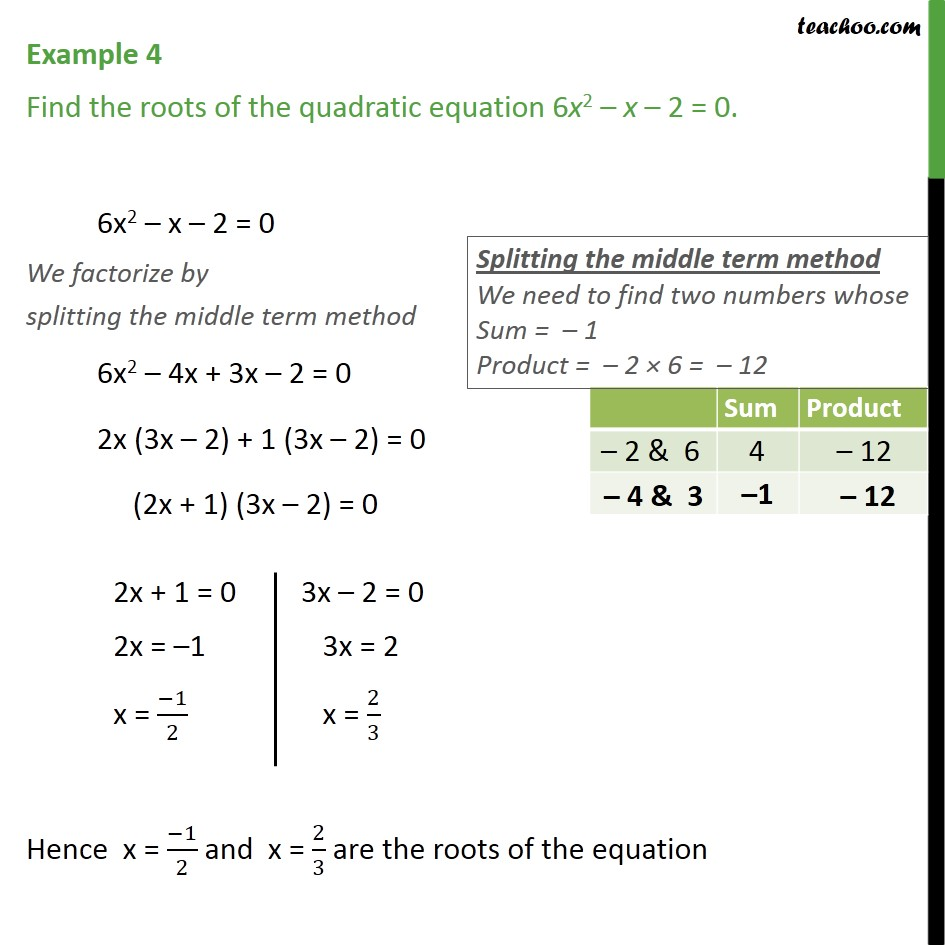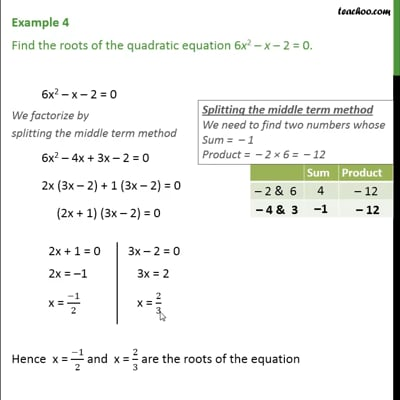Examples

Chapter 4 Class 10 Quadratic Equations (Term 2)
Serial order wiseThis video is only available for Teachoo black users

### Transcript

Example 4 Find the roots of the quadratic equation 6x2 – x – 2 = 0. 6x2 – x – 2 = 0 We factorize by splitting the middle term method We need to find two numbers whose Sum = -1 Product = -2 x 6 = -12 Checking -2 & 6, Sum = 4, Product = -12 Checking -4 & 3, Sum = -1, Product = -12 So, we take -4 and 3 6x2 – 4x + 3x – 2 = 0 2x (3x – 2) + 1 (3x – 2) = 0 (2x + 1) (3x – 2) = 0 2x + 1 =0 2x = -1 x = -1/2 3x - 2 = 0 3x = 2 x = 2/4 Hence x = (−1)/2 and x = 2/3 are the roots of the equation